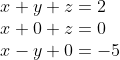## Find y

Find y:Hint
Use basic algebra to solve to solve for unknowns.
Hint 2
Use substitution to first solve for $$x$$ .
Looking at the three equations, the variable $$x$$ appears every time, meaning we should describe both the $$y$$ and $$z$$ variables in terms of $$x$$ . Analyzing the second equation:
$$x+z=0\rightarrow z=-x$$$Analyzing the third equation: $$x-y=-5\rightarrow y=x+5$$$
Substituting the new equations into the original first equation:
$$x+(x+5)+(-x)=2$$$Solving for $$x$$ : $$x=2-5=-3$$$
Since we now only have one unknown variable, we can solve for $$y$$ using the third equation:
$$y=-3+5=2$$\$
2